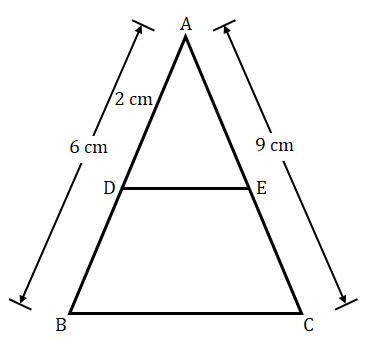"
">

# In a $Δ\ ABC$, $D$ and $E$ are points on the sides $AB$ and $AC$ respectively such that $DE\ ||\ BC$. If $AD\ =\ 2\ cm$, $AB\ =\ 6\ cm$ and $AC\ =\ 9\ cm$, find $AE$."

Given:

In a $Δ\ ABC$, $D$ and $E$ are points on the sides $AB$ and $AC$ respectively such that $DE\ ||\ BC$.

$AD\ =\ 2\ cm$, $AB\ =\ 6\ cm$ and $AC\ =\ 9\ cm$.

To do:

We have to find the measure of $AE$.

Solution:

$DE\ ||\ BC$ (given)

Therefore,

By Basic proportionality theorem,

$\frac{AD}{DB}\ =\ \frac{AE}{EC}$

This implies,

$\frac{DB}{AD}\ =\ \frac{EC}{AE}$

Adding $1$ on both sides,

$\frac{DB}{AD} + 1 = \frac{EC}{AE} + 1$

$\frac{DB+AD}{AD}=\frac{EC+AE}{AE}$

$\frac{AB}{AD}=\frac{AC}{AE}$

$\frac{6}{2}=\frac{9}{AE}$

$AE=\frac{9}{3}$

$AE=3 cm$

The measure of $AE$ is $3 cm$.

Updated on: 10-Oct-2022

30 Views